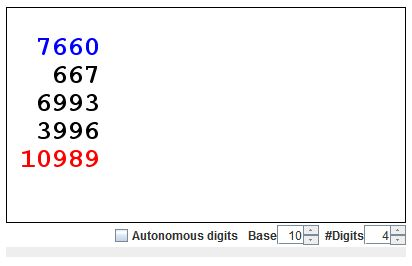# Four Digits Magic Prediction

Number 1089 invariably comes up as a result of a 2-step procedure applies to a 3-digit number. This fact serves as the basis for a baffling mathematical prediction. It is even more interesting to apply the same procedure to a 4-digit number. The result is not unique; there are 4 possibilities. However, just glancing at the original number I can tell you the result of the procedure. There are simple rules, not hard to remember; the rules underlie a spectacular mathematical trick. I am going to show how this works.

The procedure is this:

For any integer A, let A' be obtained by writing A backwards. Compute B = |A - A'| and C = B + B'.

Before reading further you may want to investigate the possible outcomes. The applet below is a calculator designed specifically for that purpose. The blue numbers are clickable. The digits increase if clicked to the right of their vertical axis, and decrease if clicked to the left of the axis.

(Note: The difference B = |A - A'| may come out with fewer than 4 digits. When this happens B is padded with the necessary number of zeros to produce a 4-digit string which then is written backwards to give B'. For example, if B = 87, B' = 7800 because the padded B is a string 0087.)

### If you are reading this, your browser is not set to run Java applets. Try IE11 or Safari and declare the site https:///www.cut-the-knot.org as trusted in the Java setup.What if applet does not run?

For 4-digit numbers, the algorithm may lead to one of 990, 9999, 10890, 10899.

A 4-digit decimal number A could be written as A = 1000a + 100b + 10c + d, giving A' = 1000d + 100c + 10b + a. Assume that a ≥ d. (If that is not true just swap A and A'.) We first consider the case where a and d are different: a > d. Then

B = 1000(a - d) + 100(b - c) + 10(c - b) + (d - a).

The key case here is when b = c so that B = 1000(a - d) + (d - a). Since d - a is negative we have to borrow from the first term:

B = 1000(a - d - 1) + 990 + (10 + d - a).

Introduce x = a - d - 1 and y = 10 + d - a. Then B = x99y and B' = y99x, with x + y = 9, making

B + B ' = 1000(x + y) + 990 + 990 + (x + y) = 9000 + 1980 + 9 = 10989.

Next, assume c > b. In this case,

B = 1000(a - d - 1) + 100(10 + b - c) + 10(c - b - 1) + (10 + d - a)

and

B' = 1000(10 + d - a) + 100(c - b - 1) + 10(10 + b - c) + (a - d - 1),

so that B + B' = 9999.

If b > c, we proceed differently:

 B = 1000(a - d) + 100(b - c) + 10(c - b) + (d - a) = 1000(a - d) + 100(b - c - 1) + 10(9 + c - b) + (10 + d - a).

Which gives B' = 1000(10 + d - a) + 100(9 + c - b) + 10(b - c - 1) + (a - d) so that

B + B' = 1000·10 + 100·8 + 10·8 + 10 = 10890.

How we obtain the last possible outcome? There is only one case remaining: a = d. If b = c, the number is palindromic and the result is 0. Otherwise, A and A' have the same thousand and unit digits, so that necessarily B is a 3-digit number ending in 0: xy0, with x + y = 9. Since, at this stage, we are dealing with 4-digit number, it needs to be padded to produce B' = yx0 (xy0 → 0xy0 → 0yx0 → yx0), giving B + B' = 990.

It looks like 4-digit numbers allow for a presentation of spectacular mental math. Ask your audience to produce a 4-digit number. If a = d, the result is either 0 or 990. If need be, read it backwards to have a > d. Then b > c gives 10890, b = c leads to 10899, and b < c will result in 9999. Not much to remember. The audience will be stunned by your ability to first subtract two 4-digit numbers and then add other two in under instantaneously.

The derivation goes through in other bases. If V and U denote the largest and the penultimate digits in some base, then the algorithm applied to 5-digit numbers may only result in 0 (for palindormic numbers), 10VUV (a > d, b = c), 10UV0 (a > d, b > c), VVVV (a > d, b < c), VV0 (a = d).1. 1089 and a Property of 3-digit Numbers
2. Four Digits Magic Prediction
3. Five Digits Magic Prediction